# Find the general solution to the differential equation dx sin χ xdy +3(y +x*) = sinx dx sin χ xdy +3(y +x*) = sinx...

Find the general solution to the differential equation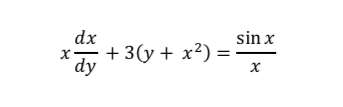dx sin χ xdy +3(y +x*) = sinx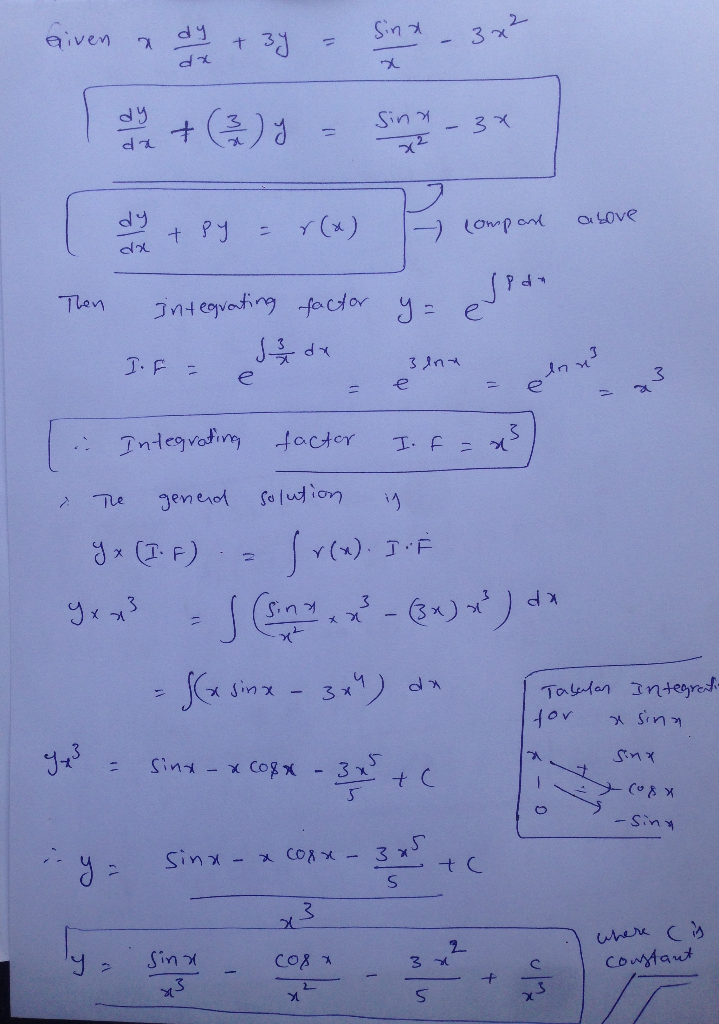##### Add Answer of: Find the general solution to the differential equation dx sin χ xdy +3(y +x*) = sinx dx sin χ xdy +3(y +x*) = sinx...
Similar Homework Help Questions
• ### QUESTION 3 Determine whether the differential equation homogeneous and find its general solution....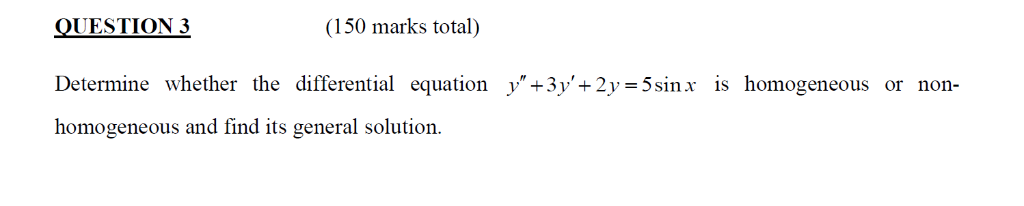2017, Q3. QUESTION 3 Determine whether the differential equation homogeneous and find its general solution. (150 marks total) y" +3 +2У = 5 sinx is homogeneous or non- QUESTION 3 Determine whether the differential equation homogeneous and find its general solution. (150 marks total) y" +3 +2У = 5 sinx is homogeneous or non-

• ### O2: 16 Marks] Find the general solution of the differential equation (sin x)y" +(cos x)y' cos x b...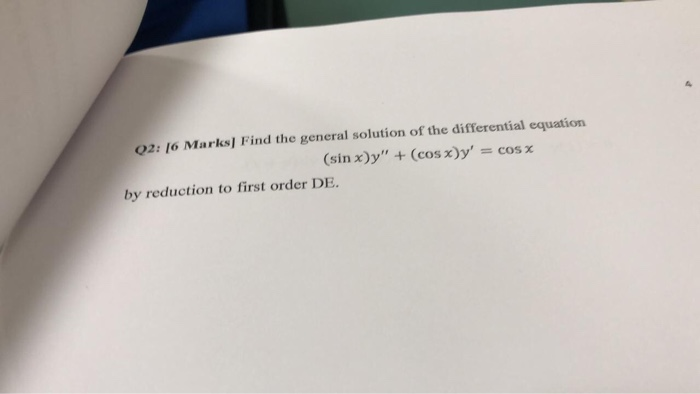o2: 16 Marks] Find the general solution of the differential equation (sin x)y" +(cos x)y' cos x by reduction to first order DE. o2: 16 Marks] Find the general solution of the differential equation (sin x)y" +(cos x)y' cos x by reduction to first order DE.

• ### (1 point) Find the indicated coefficients of the power series solution about x = 0 of the differential equation -(sinx)...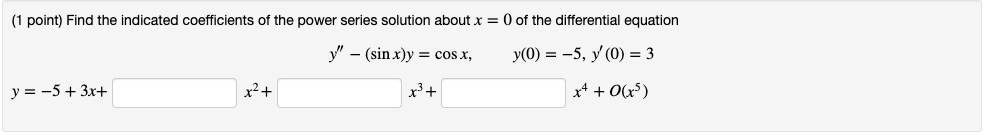(1 point) Find the indicated coefficients of the power series solution about x = 0 of the differential equation -(sinx)y y(0) = -5, y'(0) = 3 = cos x, x2 y 53x (1 point) Find the indicated coefficients of the power series solution about x = 0 of the differential equation -(sinx)y y(0) = -5, y'(0) = 3 = cos x, x2 y 53x

• ### D. Find the general solution of the differential equation56-48x + 49y - 42xy dx Give an implicit ...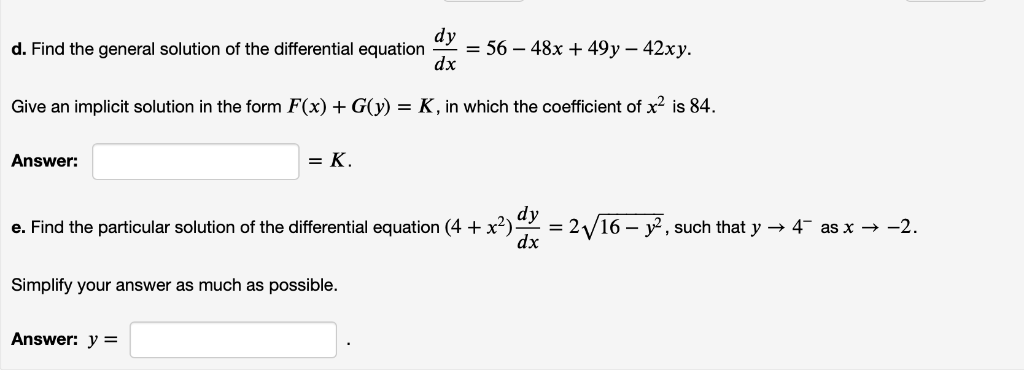d. Find the general solution of the differential equation56-48x + 49y - 42xy dx Give an implicit solution in the form F(x)GK, in which the coefficient of x2 is 84. Answer: =K e. Find the particular solution of the differential equation (4 +x2)으-2V 16-r, such that y-t as x →-2. dx Simplify your answer as much as possible. Answer: y= d. Find the general solution of the differential equation56-48x + 49y - 42xy dx Give an implicit solution in the...

• ### 5. Given the differential equation: e(dy/dx) 2x (a) Find the general solution (b) Graph particula...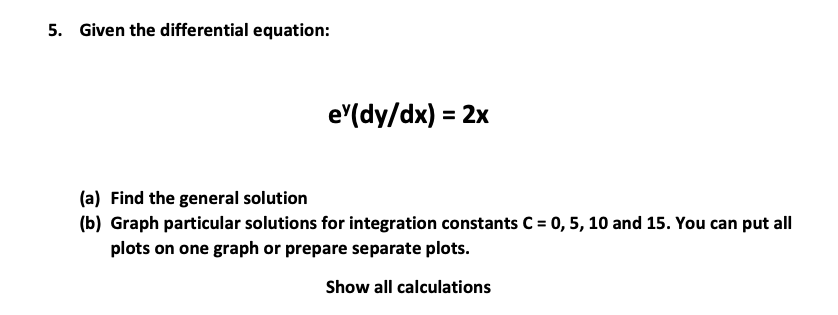5. Given the differential equation: e(dy/dx) 2x (a) Find the general solution (b) Graph particular solutions for integration constants C-0, 5, 10 and 15. You can put all plots on one graph or prepare separate plots. Show all calculations 5. Given the differential equation: e(dy/dx) 2x (a) Find the general solution (b) Graph particular solutions for integration constants C-0, 5, 10 and 15. You can put all plots on one graph or prepare separate plots. Show all calculations

• ### Question 2: (20 points) Consider the function signum Find the general global solution of the differential equation y" + (sgn x)y - 0. N.B. The general global solution is a function y: RR that is...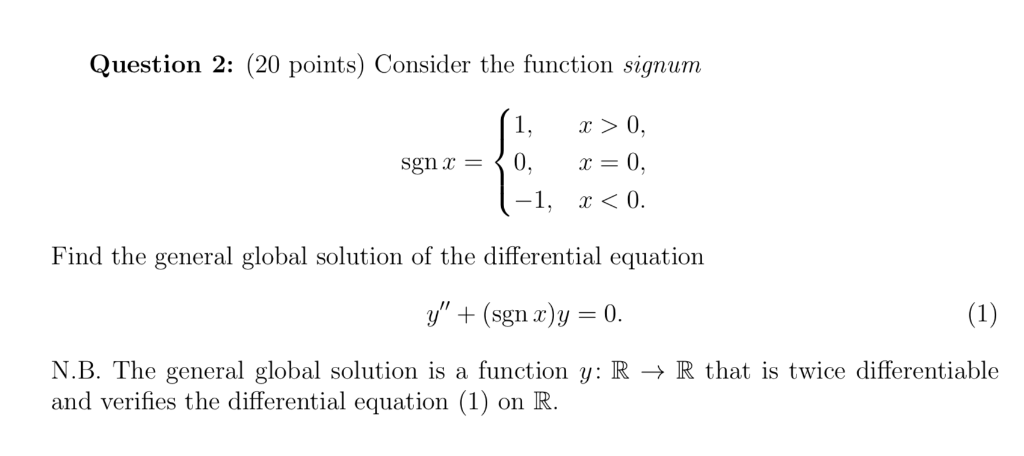Question 2: (20 points) Consider the function signum Find the general global solution of the differential equation y" + (sgn x)y - 0. N.B. The general global solution is a function y: RR that is twice differentiable and verifies the differential equation (1) on R. Question 2: (20 points) Consider the function signum Find the general global solution of the differential equation y" + (sgn x)y - 0. N.B. The general global solution is a function y: RR that is...

• ### 3. Find the general solution to the differential equation y"2y 0 as a power series about 0 involving two free const...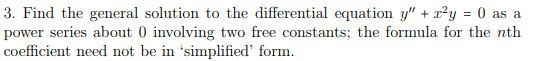3. Find the general solution to the differential equation y"2y 0 as a power series about 0 involving two free constants; the formula for the nth coefficient need not be in 'simplified' form. Show transcribed image text

• ### This is a E-Math class 3. Find the general solution of the equation (4) t y(3) = t. 3. Find the general solution of the equation (4) t y(3) = t.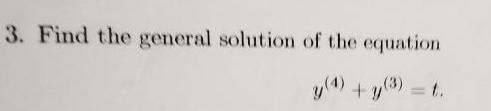This is a E-Math class 3. Find the general solution of the equation (4) t y(3) = t. 3. Find the general solution of the equation (4) t y(3) = t.

• ### dy (c) Write the first order differential equation x-y-x0 in standard form and find its general solution and then find the particular solution passing through (-2,4) (d) r (e) For what values of c do...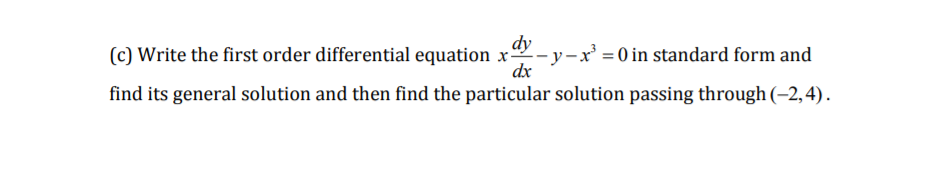dy (c) Write the first order differential equation x-y-x0 in standard form and find its general solution and then find the particular solution passing through (-2,4) (d) r (e) For what values of c does the integadx converge? al-dx converge? x(In x) O Inrlde -1 In x lux 2e Ch) In In xdr dy (c) Write the first order differential equation x-y-x0 in standard form and find its general solution and then find the particular solution passing through (-2,4) (d)...

• ### 1. Consider the Partial Differential Equation ot u(0,t) = u(r, t) = 0 a(x, 0)-x (Y), sin (! We know the general solution to the Basic Heat Equation is u(z,t)-Σ b e ). n= 1 (b) Find the unique solutio...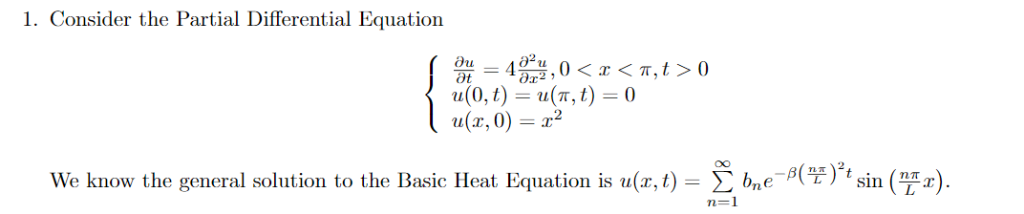1. Consider the Partial Differential Equation ot u(0,t) = u(r, t) = 0 a(x, 0)-x (Y), sin (! We know the general solution to the Basic Heat Equation is u(z,t)-Σ b e ). n= 1 (b) Find the unique solution that satisfies the given initial condition ur, 0) -2. (Hint: bn is given by the Fourier Coefficients-f(z),sin(Y- UsefulFormulas/Facts for PDEs/Fourier Series 1)2 (TiT) » x sin aL(1)1 a24(부) (TiT) 1)+1 0 1. Consider the Partial Differential Equation ot u(0,t) =...

Need Online Homework Help?